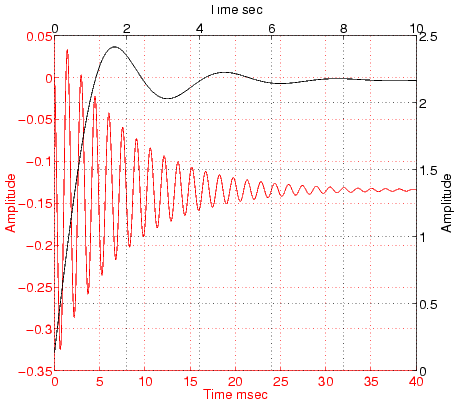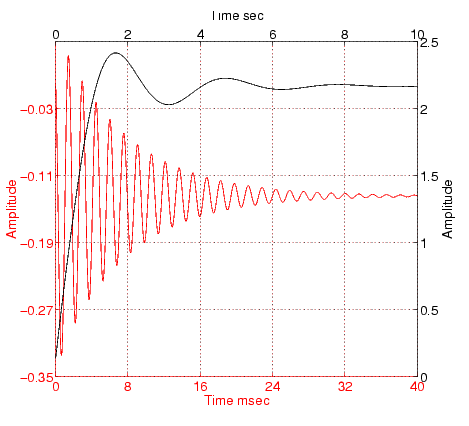GraphicsUsing Multiple X- and Y-Axes

The `XAxisLocation` and `YAxisLocation` properties specify on which side of the graph to place the x- and y-axes. You can create graphs with two different x-axes and y-axes by superimposing two axes objects and using `XAxisLocation` and `YAxisLocation` to position each axis on a different side of the graph. This technique is useful to plot different sets of data with different scaling in the same graph.

Example -- Double Axis Graphs

This example creates a graph to display two separate sets of data using the bottom and left sides as the x- and y-axis for one, and the top and right sides as the x- and y-axis for the other.

Using low-level `line` and `axes` routines allows you to superimpose objects easily. Plot the first data, making the color of the line and the corresponding x- and y-axis the same to more easily associate them.

• ```hl1 = line(x1,y1,'Color','r');
ax1 = gca;
set(ax1,'XColor','r','YColor','r')
```

Next, create another axes at the same location as the first, placing the x-axis on top and the y-axis on the right. Set the axes `Color` to `none` to allow the first axes to be visible and color code the x- and y-axis to match the data.

• ```ax2 = axes('Position',get(ax1,'Position'),...
'XAxisLocation','top',...
'YAxisLocation','right',...
'Color','none',...
'XColor','k','YColor','k');
```

Draw the second set of data in the same color as the x- and y-axis.

• ```hl2 = line(x2,y2,'Color','k','Parent',ax2);```

Creating Coincident Grids

Since the two axes are completely independent, MATLAB determines tick mark locations according to the data plotted in each. It is unlikely the gridlines will coincide. This produces a somewhat confusing looking graph, even though the two grids are drawn in different colors. However, if you manually specify tick mark locations, you can make the grids coincide.

The key is to specify the same number of tick marks along corresponding axis lines (it is also necessary for both axes to be the same size). The following graph of the same data uses six tick marks per axis, equally spaced within the original limits. To calculate the tick mark locations, obtain the limits of each axis and calculate an increment.

• ```xlimits = get(ax1,'XLim');
ylimits = get(ax1,'YLim');
xinc = (xlimits(2)-xlimits(1))/5;
yinc = (ylimits(2)-ylimits(1))/5;
```

Now set the tick mark locations.

• ```set(ax1,'XTick',[xlimits(1):xinc:xlimits(2)],...
'YTick',[ylimits(1):yinc:ylimits(2)])
```

The resulting graph is visually simpler, even though the y-axis on the left has rather odd tick mark values.Changing Axis Direction Automatic-Mode Properties© 1994-2005 The MathWorks, Inc.